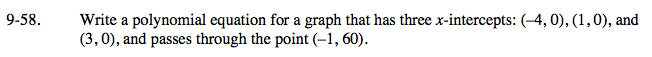### Home > A2C > Chapter Ch9 > Lesson 9.1.3 > Problem9-58

9-58.Start by writing an equation with those interceptsusing the Zero Product Property.
y = a(x + 4)(x − 1)(x − 3)

Find the stretch factor by substituting the point.

(60) = a((−1) + 4)((−1) − 1)((− 1) − 3)
60 = a(3)(−2)(−4)
60 = a(24)

$a=\frac{5}{2}$

$y=\frac{5}{2}(x+4)(x-1)(x-3)$